Next: 2.2 The Discovery of Up: 2. The Cosmic Microwave Previous: 2. The Cosmic Microwave

# 2.1 Prologue: Black Bodies

The Cosmic Microwave Background is nature's most perfect realization of ideal Blackbody Radiation. To interpret the CMB, we need to understand what this means.

Everything shines like a star; not as much, of course, but in the same way. Stars shine because they are hot -- anything hot shines; we call this thermal radiation. How hot do you have to be? Above absolute zero, that's all. And that's everything (third law of thermodynamics: nothing can be cooled to absolute zero).

In a cavity (e.g. an oven) with opaque walls all at the same temperature, emission and absorption of photons will eventually come into balance. Then it turns out that in any small region we will find statistically equal numbers of photons travelling in any direction, with the number at each frequency depending only on the temperature. In other words, the temperature fixes

• the total energy per unit volume, or energy density

u = aT4

where a is the radiation constant;
• the amount of radiation heading in any direction, i.e. the intensity (c.f. Section 1.2.8):

I =aT4

• the shape of the spectrum; for instance quantified by the intensity per unit frequency I(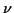), which is equal to

B(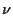, T)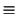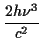The constants h and kB belong to Planck and Boltzmann, respectively. This is the Planck spectrum, also called, for reasons about to be explained, the black body spectrum (Fig. 2.1).Although photons with all frequencies are present in the Planck spectrum, most have energies hclose to 3kBT (in general, the energy per particle in anything at temperature T is roughly kBT).

If you are not in a constant-temperature oven, how much you shine (your intensity or surface brightness) depends on three things: your temperature, how easy it is for photons to go through your surface rather than bounce off (your albedo), and your transparency. We quantify transparency by optical depth,: defined so that e-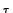is the probability that a photon will pass right through you rather than be absorbed. We have already met this concept in the rather different context of gravitational lensing. Objects with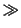1 are said to be optically thick.

Let's put a partially transparent slab in our oven and look through it at the oven walls. A fraction (1 - e-) of the radiation entering from behind is absorbed; but in thermal equilibrium the net radiation must be unchanged, so the slab must emit with a brightness

(1 - e-)B(, T)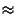B(, T).

The approximation holds if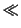1. The emission depends on the temperature of the slab, but not on the fact that it is in an oven, so this is the general formula for thermal emission. Albedo and optical depth usually depend on the frequency of the photon; for instance, people are optically thick to visible light but are fairly transparent to X-rays. An object with albedo zero and infinite optical depth at all frequencies would absorb every photon that reached it, and, by the same token, it would emit a radiation with a pure Planck spectrum. This is called a black body.

A few astronomical objects have spectra which are very roughly given by black bodies, such as stars and planets (Fig. 2.2). But the spectra are more complicated than ideal black bodies for many reasons. For instance, the Sun contains many layers with different temperatures, which are more or less transparent at different frequencies. We see a narrow absorption line when a cool outer layer that is usually transparent becomes partially opaque at a particular frequency, so that the brightness near that frequency drops towards the value for the cool layer instead of the hot surface beneath. Similarly, the corona is a hot layer that is mostly transparent, but shows bright emission lines at particular X-ray frequencies where the optical depth is relatively high.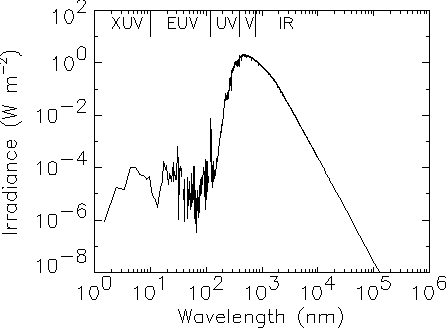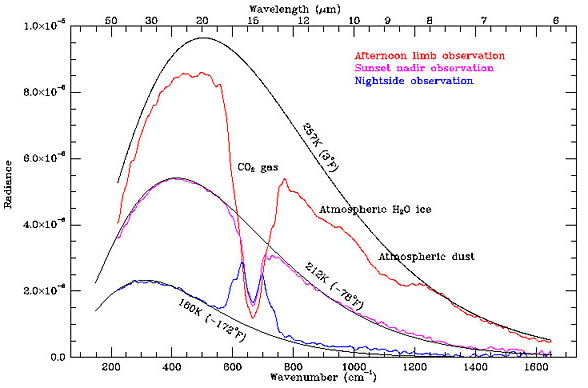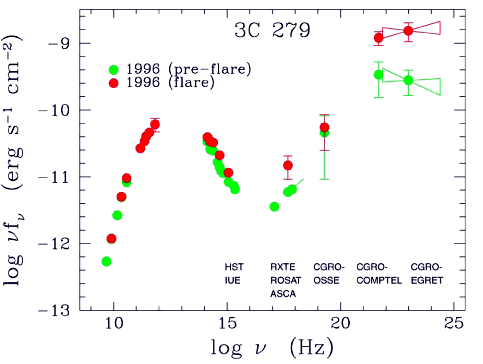As the name suggests, it takes a lot of stuff to generate a high optical depth, especially across a wide range of frequencies rather than in a narrow line. So it is not surprisingly that space is usually very transparent (of course stars and planets fill a negligible volume of space).

## 2.1.2 Temperature and Brightness

The formal SI units for surface brightness or intensity I() are watts per hertz per steradian per square metre. As well as being a mouthful, many astronomers still work with c.g.s. units which can cause confusion. It is more convenient to quote the Planck brightness temperature, TPB, that is, the temperature which would give the required intensity according to the Planck spectrum:

B(, TPB)I()

Usually there is no connection between TPB and the physical temperature of the emitting object, since real black bodies are very hard to find.

In radio astronomy it is more common to use a variant form of brightness temperature which, instead of the Planck law, uses the Rayleigh-Jeans law,

BRJ(, T) =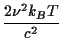The Rayleigh-Jeans brightness BRJ(, T) is a good approximation to the Planck brightness B(, T) at frequencies low enough that hkBT. Unqualified brightness temperature TB means the Rayleigh-Jeans version.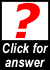Use the approximation ex1 + x which applies for x1 to show that BRJ(, T)B(, T) if hkBT.

Use the approximation to simplify the denominator in the formula for the black body curve:

exp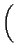- 1= 1 +- 1 =Hence

B(, T)2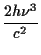==This is the formula for BRJ.

## 2.1.3 Effect of Redshift

As we follow black body radiation over cosmic time we must deal with the redshift. This is very easy, because a black body spectrum with temperature T at redshift z simply turns into a new black body spectrum with a cooler temperature T' = T/(1 + z).

This result is not too difficult to derive. As redshifting just stretches the spectrum, the shape will be preserved, but the peak wavelength will be longer by a factor of (1 + z). We saw that in a black body,

h= hc/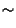kBT

so the redshifted peak will indeed correspond to T/(1 + z). We also have to show that the amplitude of the spectrum, not just the shape, corresponds to the new temperature. This follows from the result we found in Section 1.2.8, that observed intensity suffers cosmological dimming by a factor of (1 + z)4. The observed intensity of a Black Body spectrum emitted with temperature T at redshift z will therefore be

I' =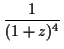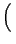aT4=a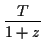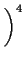=aT'4,

as required.

Finally, if photons are travelling in equal numbers and with the same distribution of energies in all directions (i.e. the intensity is isotropic), then the energy density in photons is

u(T) =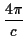I(T).

Imagine enclosing such a photon "gas" in a totally reflecting box. If the box is smoothly expanded by a factor a in each dimension, the energy density drops in such a way that we still have u(T') = 4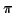I(T')/c but with a new T' = T/a. The same applies to the universe, where a = 1 + z is the expansion factor between redshift z and now; as we might expect, the redshift effect on the CMB intensity is equivalent to the expansion effect on its energy density. By the way, this corresponds to u(T)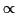(1 + z)4R-4, as we derived for radiation (with any spectrum) in Section 1.3.2.

## 2.1.4 The temperature of space

In 1917 the French astrophysicist Charles Fabry published a quirky paper which nicely illustrated some concepts related to black body radiation. What, asked Fabry, is the temperature of empty space? That is, if an object was placed in space, what temperature would it settle down at? Clearly, it would absorb starlight, and also emit its own thermal radiation. The equilibrium temperature would be the one where the energy gain from absorption was equal to the loss from emission. Fabry's point was that there was no well-defined answer to his question: the equilibrium temperature would depend on the physical properties of the object, much more than on the amount of starlight in space which one might have thought would define some sort of effective temperature.

For instance, if the object was a black body it would absorb all incident photons and so the equivalent temperature would be that of a black body cavity containing the same radiation density as space. For interstellar space in our Galaxy he calculated this to be 3o absolute, or 3 K in modern notation. But unlike a black body cavity, the spectrum arriving at the object would be very different from the one leaving it: starlight is in the visible band (wavelength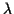500 nm), while the Planck spectrum at 3 K peaks at1 mm, on the border between the radio and the infrared. Roughly speaking, starlight has the spectral shape of a black body at around 6000 K, but an intensity 1013 times less, essentially because only about one part in 1013 of the sky is actually covered by the surfaces of stars (for a position inside a galaxy; the value is far smaller in intergalactic space).

Now suppose the object was good at absorbing visible light but had a high albedo near1 mm, so that it radiated much less than a black body at that wavelength. The incoming energy would heat it until eventually emission matched absorption despite the poor radiative efficiency (we now call this the Greenhouse Effect). If absorption and emission were confined to only a single wavelength in the visible band, the body would have to shine in visible light to get rid of its excess heat; the equilibrium temperature would be the Planck brightness temperature at the wavelength of absorption, which is around about a thousand degrees in the visible band in interstellar space. Atoms and simple molecules do absorb at only a small number of discrete wavelengths, and so they can reach very high excitation temperatures in interstellar space (which is why they are often ionised). By the same token, if the object was a poor absorber of visible light and a good radio emitter, it could cool to whatever the radio brightness temperature was; almost negligibly small, as far as Fabry knew.

Fabry's argument was included in Arthur Eddington's famous book The Internal Constitution of the Stars, and in this form was transmitted to later generations of astronomers. Eddington made the point in a slightly different way, choosing to define the temperature of space as the equivalent black-body temperature, 3 K, and only later showing by example that this was a fairly meaningless number. This re-arrangement had a disastrous effect, as we will see, because casual readers tended to pick up only the first part of the argument.

Next: 2.2 The Discovery of Up: 2. The Cosmic Microwave Previous: 2. The Cosmic Microwave